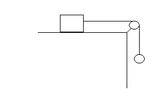# Friction conceptual question

Imagine a pulley system, where a puck is connected by a massless string to a massless pulley that joins a mass m thats hanging at the end of the table.

Would the force of friction change as you increase mass m , the object thats hanging? why so?

Imagine a pulley system, where a puck is connected by a massless string to a massless pulley that joins a mass m thats hanging at the end of the table.

Would the force of friction change as you increase mass m , the object thats hanging? why so?

Didn't quite understand what you meant by mass m 'hanging' at the end of the table.
Is it something like this?The mass m on the table and the puck hanging?

Or is it just the other way, the puck on the table and the mass hanging?

exactly. So I have a question. Would the force of friction increase as the block thats on the table, mass increases?

exactly. So I have a question. Would the force of friction increase as the block thats on the table, mass increases?
That depends on the mass of the puck. We're talking about static friction here, which is a self-adjusting force. The frictional force acting on the block depends on mass of the puck.

If the mass of the puck was gradually increasing, then what would happen to the friction.

Its like this. You go on increasing the mass of the puck. the weight of the puck increases accordingly. The friction between the block adjusts itself to a value that equals the weight of the puck UNTIL the point of limiting friction (max. value of static friction). The mass of the puck at this point is the greatest mass it can have for the system to be in equilibrium. If you increase the mass of the puck slightly, there is a net acceleration.
Let the mass of block and puck be M and m
Writing equations, mg - T = 0 and T - f =0 (If you consider the acceleration of both the bodies to be zero)
We get, mg = f.
Mass of the puck is proportional to the frictional force on the block. Hope this makes things clear..

So what if the mass of the hanger was constant and the mass of the block was constantly increasing, what would happen to friction?

would it stay constant because the tension that the rope is providing is constant.

So what if the mass of the hanger was constant and the mass of the block was constantly increasing, what would happen to friction?

would it stay constant because the tension that the rope is providing is constant.

Yea, exactly. You can go on increasing the mass of the block but the friction force remains constant.

All this, provided..the mass of the puck < mass of the block. Unless, this condition is satisfied there will be a net acceleration and friction will be different.

and if the block had a constant mass and the hanger had a gradually increasing mass the force of friction would increase up until a point?

and if the block had a constant mass and the hanger had a gradually increasing mass the force of friction would increase up until a point?

Yes, it would increase till limiting friction is experienced by the block. Further increase would lead to the block accelerating to the right.

thanks for making it clear